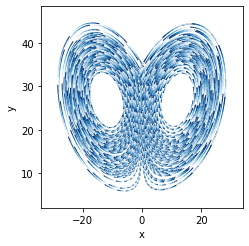# Lorenz Attractor Example#

URL: http://bokeh.pydata.org/en/latest/docs/gallery/lorenz.html

Most examples work across multiple plotting backends, this example is also available for:

```import numpy as np
import holoviews as hv
hv.extension('matplotlib')
```## Declare the data#

```from scipy.integrate import odeint

sigma = 10
rho = 28
beta = 8.0/3
theta = 3 * np.pi / 4

def lorenz(xyz, t):
x, y, z = xyz
x_dot = sigma * (y - x)
y_dot = x * rho - x * z - y
z_dot = x * y - beta* z
return [x_dot, y_dot, z_dot]

initial = (-10, -7, 35)
t = np.arange(0, 100, 0.006)

solution = odeint(lorenz, initial, t)

x = solution[:, 0]
y = solution[:, 1]
z = solution[:, 2]
xprime = np.cos(theta) * x - np.sin(theta) * y

paths = zip(np.array_split(xprime, 7), np.array_split(z, 7))
lorenzian = hv.Path([{('x', 'y'): np.array(d).T, 'index': i}
for i, d in enumerate(paths)], vdims='index')
```

## Plot#

```lorenzian.opts(color='index', cmap='Blues', linewidth=1)
```This web page was generated from a Jupyter notebook and not all interactivity will work on this website. Right click to download and run locally for full Python-backed interactivity.